Open In App
Related Articles

# Image formation by Spherical Lenses

You might have used a microscope in the science lab for magnifying the micro-size object. It basically magnifies tiny objects and we can see the enlarged image of that object. Telescopes are used by scientists to the planets and stars which are far- far away from the earth. You might see the spectacles used by old people. The glass used in those spectacles is thick. These are examples of lenses. The image formed by the lens can be smaller or larger. The size of the image depends upon the type of lens that is being used. It also depends upon how far the object is placed in front of the lens. We will discuss all in this article.

A piece of transparent glass bounded by two surfaces, at least one of which is a curved surface, which concentrates or disperses the light rays when passes through them by refraction is called the lens.

### Types of Lenses

• Concave lens: The lens which is thicker at the end than the middle is called the concave lens. It is also called diverging lens as it spreads out the light rays that have been refracted through it. It has the ability to diverge the parallel beam of light.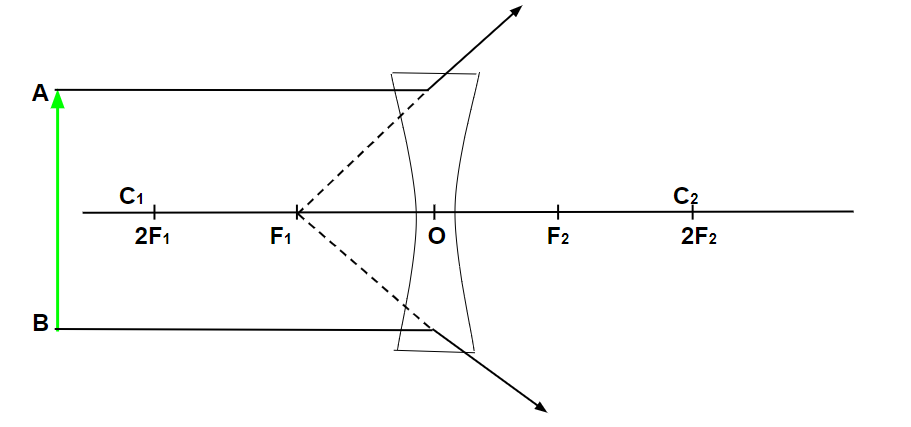A diverging lens (Concave lens)

• Convex lens: The lens which is thicker at the middle than the end is called a convex lens. It is also called a converging lens as it converges the parallel beam of light into a point.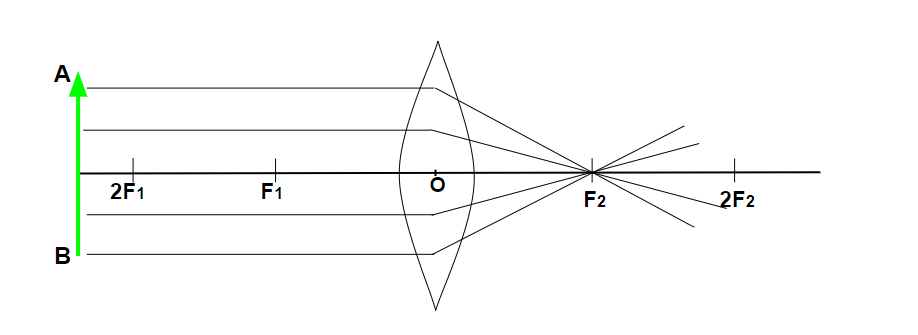A Converging lens (Convex lens)

### Terminologies related to Spherical Lens

• Pole (p): It is the middle point of the spherical lens or mirror.
• Centre of curvature (C): It is the centre of the sphere from which the mirror is formed.
• Principal axis: It is the lines passing through the pole and the centre of curvature of the lens.
• Principal focus (F): It is the point at which a narrow beam of light converges or diverges.
• Focal length (f): It is the distance between the focus and the poles of the mirror.

### Image formed by the Convex Lens

There are six different cases for the image formation by a convex lens, which are discussed as:

When an object is at infinity:

When object AB (shown in the figure below) is placed at infinity that is behind the 2F1 of the convex mirror, the image formed after the refraction will on focus F2 which is on the opposite side of the convex lens. The size of the image is smaller than the object and the image will be real and inverted(i.e upside down and downside up).

• The image formed at – Focus (F2)
• The nature of the image formed – Real and inverted
• The size of the image formed – Diminished (smaller)When an object is at infinity, the image is formed at Focus (F2).

When an object is placed behind the Centre of Curvature (C1):

When the object is placed behind the centre of curvature (C1) or behind Focus (2F1) of the convex lens, the image formed after the refraction will be between the foci of another side of the lens (i.e. F2 and 2F2). The size of the image is smaller than the object. The nature of the image will be real (can be seen on the screen) and inverted( upside down).

• The image formed at – Between 2F2 and F2.
• The nature of the image formed – Real and inverted
• The size of the image formed – Diminished (smaller)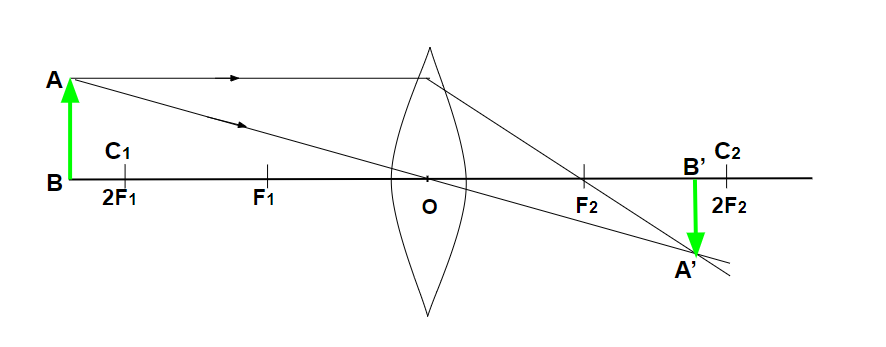When an object is placed behind the Centre of Curvature (C1), the image is formed between 2F2 and F2.

When the object is placed at the centre of curvature (C1 or 2F1):

When an object is placed at the centre of curvature (C1) or focus (2F1) of the convex lens, the image formed after the refraction will be on the centre of curvature (C2) or focus (2F2) on the other side of the lens. The size of the image is the same as the size of the object. The nature of the image is real and inverted.

• The image formed at – C2 or 2F2.
• The nature of the image formed – Real and inverted
• The size of the image formed – Equal to the object size.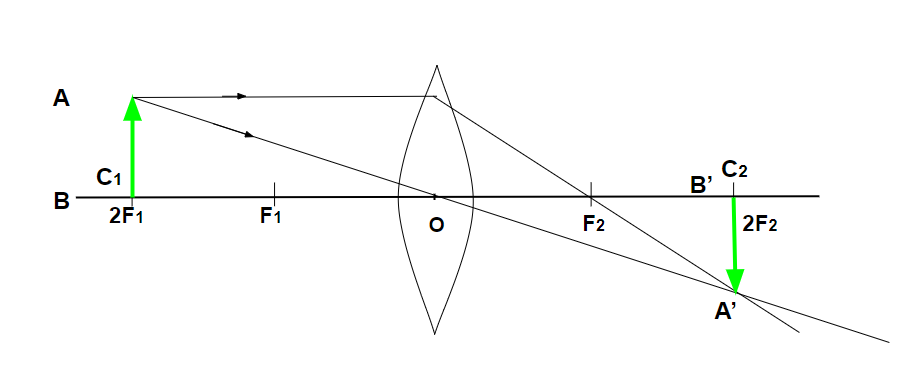When the object is placed at the centre of curvature (C1 or 2F1), the image is formed at C2 or 2F2.

When the object is placed between 2F1 and F1:

When an object is placed between the centre of curvature and the focus (F1) of the convex lens, the image formed after reflection will be behind the centre of curvature (C2). The size of the image will be greater than the object. The nature of the image will be real and inverted.

• The image formed at – Behind centre of curvature (C2)
• The nature of the image formed – Real and inverted
• The size of the image formed – Enlarged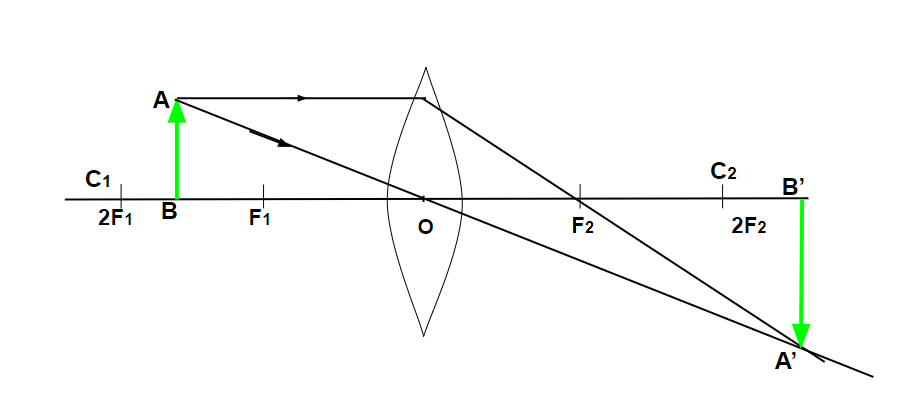When the object is placed between 2F1 and F1, the image is formed behind the centre of curvature (C2).

When the object is placed at focus (F1):

When an object is placed at focus (F1) of a convex lens. The image formed after reflection will be at infinity (opposite side of the lens). The size of the object will be much larger than the object. The nature of the image will be real and inverted.

• The image formed at – Infinity (opposite side of the object)
• The nature of the image formed – Real and inverted
• The size of the image formed – Enlarged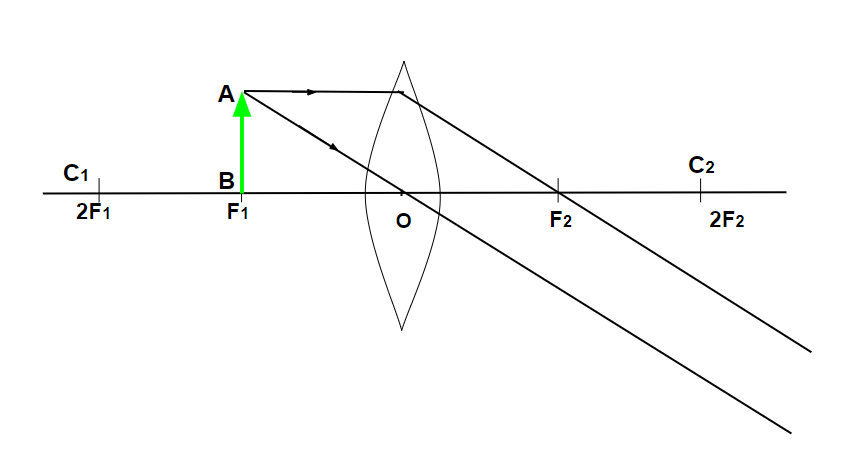When the object is placed at focus (F1), the image formed is at Infinity (opposite side of the object).

When the object is placed between pole and focus (O and F1):

When the object is placed between the focus (F1) and the optic centre (O) of the convex lens. The image is formed at the same side of the object behind the centre of curvature (C) or focus (F1) of the lens. The size of the image will be larger than the object. The nature of the image will be Virtual Erect.

• The image formed at – At the same side of the object behind 2F2.
• The nature of the image formed – Virtual and Erect.
• The size of the image formed – Enlarged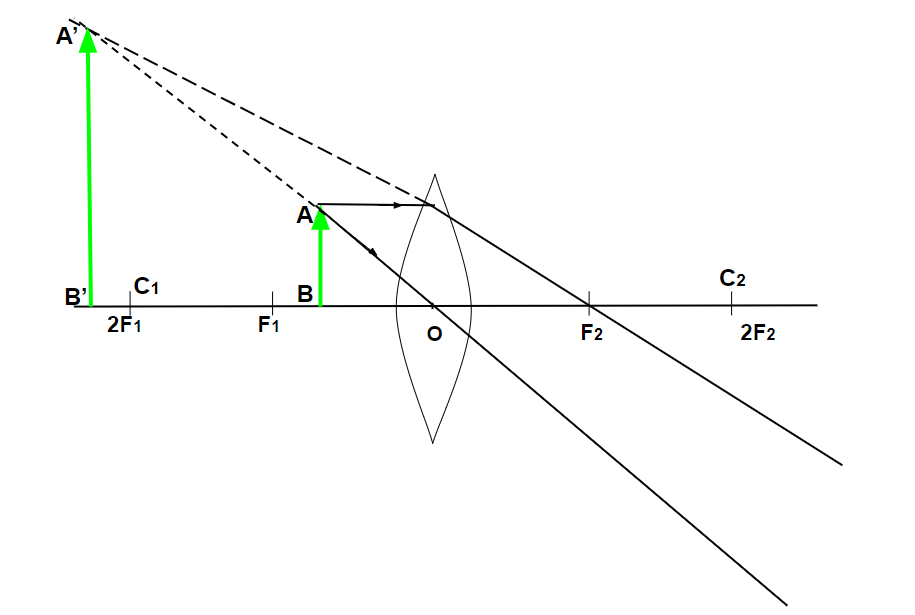When the object is placed between pole and focus (O and F1), the image formed is at the same side of the object behind 2F2.

### Image formed by Concave lens

There are only two different cases for the image formation by a concave lens, which are discussed as:

When the object is placed at infinity:

When an object is placed at infinity of the concave lens (shown below). The image formed after refraction will be at the focus (F1) on the same side of the object. The size of the image will be much smaller than the object. The nature of the image will be virtual and erect.

• The image formed at – Focus (F1)
• The nature of the image formed – Virtual and Erect
• The size of the image formed – Highly diminishedWhen the object is placed at infinity, the image formed is at focus (F1).

When the object is placed at a finite distance from the lens:

When the object is placed at any finite distance in front of the concave lens. The image formed after refraction will be between the optic centre (O) and the focus (F) of the concave lens. The size of the image will be smaller than the object.

• The image formed at – Between F1 and optical centre
• The nature of the image formed – Virtual and Erect
• The size of the image formed – Diminished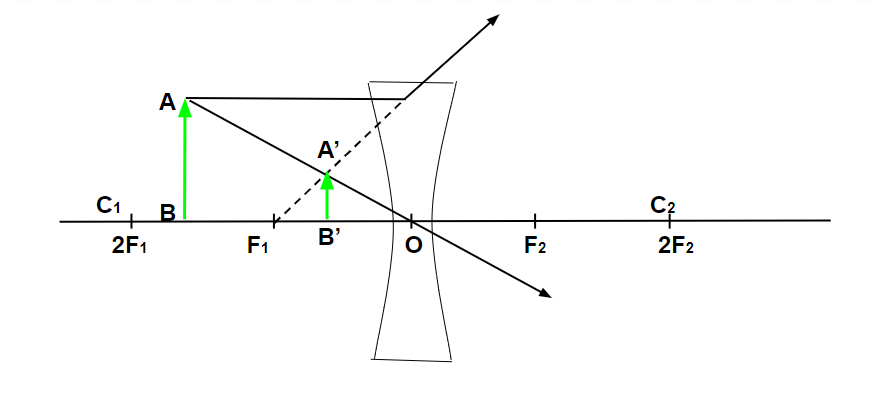When the object is placed at a finite distance from the lens, the image formed is between F1 and the optical centre.

### Sample Questions

Question 1: What is the real image?

The image formed when rays of light meet at a certain point after reflection/refraction is real image. Real images can be displayed on screen.

Question 2: What is a virtual image?

The image formed when rays of light appear to meet at particular point is called virtual image.

Question 3: What is a ray diagram?

The type of diagram which helps to trace the path that light takes in order for a person to view a point on the image of an object is called a ray diagram.

Question 4: What will be the focal length of a lens, if the radius is 16 cm?

The focal length is half of the radius of lens, i.e.

f= R / 2

= 16 cm / 2

= 8 cm

Therefore, the focal length will be 8 cm.

Question 5: What will be the focal length of a lens when it is cut along the principal axis?